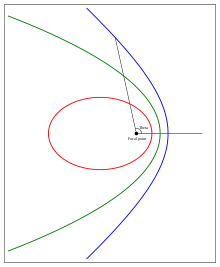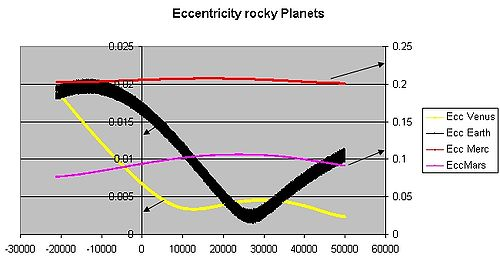# Orbital eccentricity

﻿
Orbital eccentricityAn elliptic Kepler orbit with an eccentricity of 0.7 (red), a parabolic Kepler orbit (green) and a hyperbolic Kepler orbit with an eccentricity of 1.3 (blue)

The orbital eccentricity of an astronomical body is the amount by which its orbit deviates from a perfect circle, where 0 is perfectly circular, and 1.0 is a parabola, and no longer a closed orbit. It derives its name from the parameters of conic sections, as every Kepler orbit is a conic section.

## Definition

In a two-body problem with inverse-square-law force, every orbit is a Kepler orbit. The eccentricity of this Kepler orbit is a positive number that defines its shape.

The eccentricity may take the following values:

• circular orbit:$e=0\,\!$
• elliptic orbit:$0 (see Ellipse)
• parabolic trajectory:$e=1\,\!$ (see Parabola)
• hyperbolic trajectory:$e>1\,\!$ (see Hyperbola)

The eccentricity e is given by$e = \sqrt{1 + \frac{2 E L^{2}}{m_\text{red} \alpha ^{2}}}$

where E is the total orbital energy, L is the angular momentum, mred is the reduced mass. and α the coefficient of the inverse-square law central force such as gravity or electrostatics in classical physics:$F = \frac{\alpha}{r^{2}}$

(α is negative for an attractive force, positive for a repulsive one) (see also Kepler problem).

or in the case of a gravitational force:$e = \sqrt{1 + \frac{2 \epsilon h^{2}}{\mu^2}}$

where$\epsilon$ is the specific orbital energy (total energy divided by the reduced mass), μ the standard gravitational parameter based on the total mass, and h the specific relative angular momentum (angular momentum divided by the reduced mass).

For values of e from 0 to 1 the orbit's shape is an increasingly elongated (or flatter) ellipse; for values of e from 1 to infinity the orbit is a hyperbola branch making a total turn of 2 arccsc e, decreasing from 180 to 0 degrees. The limit case between an ellipse and a hyperbola is for e precisely 1 which is parabola.

Radial trajectories are classified as elliptic, parabolic, or hyperbolic based on the energy of the orbit, not the eccentricity. Radial orbits have zero angular momentum and hence eccentricity equal to one. Keeping the energy constant and reducing the angular momentum, elliptic, parabolic, and hyperbolic orbits each tend to the corresponding type of radial trajectory while e tends to 1 (or in the parabolic case: remains 1).

For a repulsive force only the hyperbolic trajectory, including the radial version, is applicable.

For elliptical orbits, a simple proof shows that arcsin(e) yields the projection angle of a perfect circle to an ellipse of eccentricity e. For example, to view the eccentricity of the planet Mercury (e=0.2056), one must simply calculate the inverse sine to find the projection angle of 11.86 degrees. Next, tilt any circular object (such as a coffee mug viewed from the top) by that angle and the apparent ellipse projected to your eye will be of that same eccentricity.

## Etymology

From Medieval Latin eccentricus, derived from Greek ekkentros "out of the center", from ek-, ex- "out of" + kentron "center". Eccentric first appeared in English in 1551, with the definition "a circle in which the earth, sun. etc. deviates from its center." Five years later, in 1556, an adjective form of the word was added.

## Calculation

Eccentricity of an orbit can be calculated from orbital state vectors as a magnitude of eccentricity vector:$e= \left | \mathbf{e} \right |$

where:

•$\mathbf{e}\,\!$ is eccentricity vector.

For elliptical orbits it can also be calculated from distance at apoapsis and periapsis:$e={{r_a-r_p}\over{r_a+r_p}}$$=1-\frac{2}{(r_a/r_p)+1}$

where:

•$r_a\,\!$ is radius at apoapsis (i.e., the farthest distance of the orbit to the center of mass of the system, which is a focus of the ellipse).
•$r_p\,\!$ is radius at periapsis (the closest distance).

The eccentricity of an elliptical orbit can also be used to obtain the ratio of the periapsis to the apoapsis:${{r_p}\over{r_a}}={{1-e}\over{1+e}}$

## ExamplesGravity Simulator plot of the changing orbital eccentricity of Mercury, Venus, Earth, and Mars over the next 50,000 years. The 0 point on this plot is the year 2007.

The eccentricity of the Earth's orbit is currently about 0.0167, meaning that the Earth's orbit is nearly circular. Over hundreds of thousands of years, the eccentricity of the Earth's orbit varies from nearly 0.0034 to almost 0.058 as a result of gravitational attractions among the planets (see graph).

In other values, Mercury (with an eccentricity of 0.2056) holds the title as the largest value among the planets of the Solar System. Prior to the redefinition of its planetary status, the dwarf planet Pluto held this title with an eccentricity of about 0.248. The Moon also holds a notable value at 0.0549. For the values for all planets in one table, see List of gravitationally rounded objects of the Solar System.

Most of the solar system's asteroids have eccentricities between 0 and 0.35 with an average value of 0.17. Their comparatively high eccentricities are probably due to the influence of Jupiter and to past collisions.

The eccentricity of comets is most often close to 1. Periodic comets have highly eccentric elliptical orbits, with eccentricities just below 1; Halley's Comet's elliptical orbit, for example, has a value of 0.967. Non-periodic comets follow near-parabolic orbits and thus have eccentricities very close to 1. Examples include Comet Hale-Bopp with a value of 0.995 and comet C/2006 P1 (McNaught) with a value of 1.000019. As Hale-Bopp's value is less than 1, its orbit is elliptical and so the comet will in fact return. Comet McNaught has a hyperbolic orbit while within the influence of the planets, but is still bound to the Sun with an orbital period of about 100,000 years. As of a 2010 Epoch, Comet C/1980 E1 has the largest eccentricity of any known hyperbolic comet with an eccentricity of 1.057, and will leave the solar system indefinitely.

Planet Neptune's largest moon Triton has an eccentricity of 0.000016 which is the smallest eccentricity of any known body in the solar system; its orbit is as close to a perfect circle as can be currently measured.

## Mean eccentricity

The mean eccentricity of an object is the average eccentricity as a result of perturbations over a given time period. Neptune currently has an instant (current Epoch) eccentricity of 0.0113, but from 1800 A.D. to 2050 A.D. has a mean eccentricity of 0.00859.

## Climatic effect

Orbital mechanics require that the duration of the seasons be proportional to the area of the Earth's orbit swept between the solstices and equinoxes, so when the orbital eccentricity is extreme, the seasons that occur on the far side of the orbit (aphelion) can be substantially longer in duration. Today, northern hemisphere fall and winter occur at closest approach (perihelion), when the earth is moving at its maximum velocity. As a result, in the northern hemisphere, fall and winter are slightly shorter than spring and summer. In 2006, summer was 4.66 days longer than winter and spring was 2.9 days longer than fall.[citation needed] Axial precession slowly changes the place in the Earth's orbit where the solstices and equinoxes occur. Over the next 10,000 years, northern hemisphere winters will become gradually longer and summers will become shorter. Any cooling effect, however, will be counteracted by the fact that the eccentricity of Earth's orbit will be almost halved[citation needed], reducing the mean orbital radius and raising temperatures in both hemispheres closer to the mid-interglacial peak.

Wikimedia Foundation. 2010.

### Look at other dictionaries:

• Orbital inclination change — is an orbital maneuver aimed at changing the inclination of an orbiting body s orbit. This maneuver is also known as an orbital plane change as the plane of the orbit is tipped. This maneuver requires a change in the orbital velocity vector… …   Wikipedia

• Orbital forcing — is the effect on climate of slow changes in the tilt of the Earth s axis and shape of the orbit (see Milankovitch cycles). These orbital changes change the total amount of sunlight reaching the Earth by up to 25% at mid latitudes (from 400 to 500 …   Wikipedia

• Eccentricity — may refer to: * Eccentricity (behavior), unusual or odd behavior on the part of a person, as opposed to being normal * Eccentricity (mathematics), a parameter associated with every conic section * Eccentricity vector * Eccentricity (graph theory) …   Wikipedia

• Orbital resonance — For the science fiction novel by John Barnes, see Orbital Resonance (novel). In celestial mechanics, an orbital resonance occurs when two orbiting bodies exert a regular, periodic gravitational influence on each other, usually due to their… …   Wikipedia

• Orbital mechanics — A satellite orbiting the earth has a tangential velocity and an inward acceleration. Orbital mechanics or astrodynamics is the application of ballistics and celestial mechanics to the practical problems concerning the motion of rockets and other… …   Wikipedia

• Eccentricity (mathematics) — In mathematics, the eccentricity, denoted e or varepsilon, is a parameter associated with every conic section. It can be thought of as a measure of how much the conic section deviates from being circular.In particular, * The eccentricity of a… …   Wikipedia

• Orbital elements — are the parameters required to uniquely identify a specific orbit. In celestial mechanics these elements are generally considered in classical two body systems, where a Kepler orbit is used (derived from Newton s laws of motion and Newton s law… …   Wikipedia

• Orbital perturbation analysis (spacecraft) — Isaac Newton in his Philosophiæ Naturalis Principia Mathematica demonstrated that the gravitational force between two mass points is inversely proportional to the square of the distance between the points and fully solved corresponding two body… …   Wikipedia

• Orbital station-keeping — In astrodynamics orbital station keeping is a term used to describe the orbital maneuvers made by thruster burns that are needed to keep a spacecraft in a particular assigned orbit. For many Earth satellites the effects of the non Keplerian… …   Wikipedia

• Orbital speed — Not to be confused with Escape Velocity. The orbital speed of a body, generally a planet, a natural satellite, an artificial satellite, or a multiple star, is the speed at which it orbits around the barycenter of a system, usually around a more… …   Wikipedia